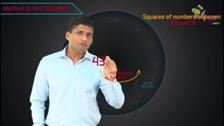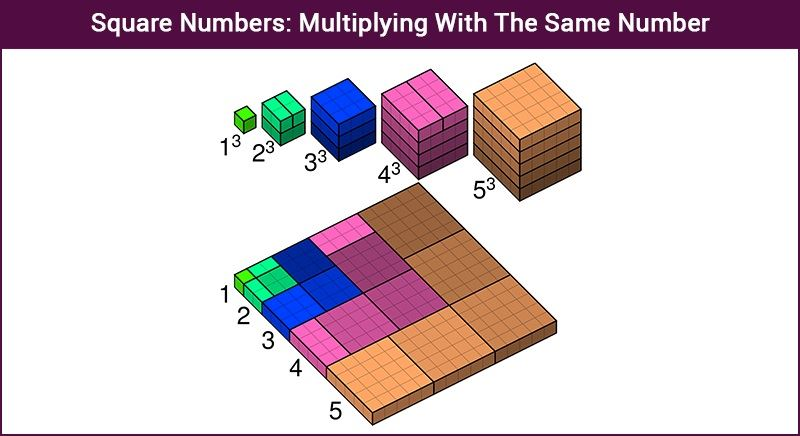# Square Numbers: Multiplying With The Same Number## What are Square Numbers?

When a number is multiplied by itself, the resultant is called a ‘Square Number’.

In geometry, a square has all its sides equal. Therefore the area of the square is equal to the square of its side.

Area of a square = Side $\times$ Side
Therefore, we can say;
Square number = a $\times\; a \; = a^{2}$

Hence, area of square is best example of square of a number.Side of square (in cm) Area of square (in cm2) 3 3 × 3 = $3^{2}$ = 9 5 5 × 5 = $5^{2}$ = 25 7 7 × 7 = $7^{2}$ = 49 8 8 × 8 = $8^{2}$ = 64

Numbers such as 1, 4, 9, 16, 25, 36, 49, 64, etc. are special numbers as these are the product of a number by itself.

If we express a number (x) in terms of the square of any natural number such as a2, then x is a square number. For example, 100 can be expressed as 10 × 10 = 102, where 10 is a natural number, therefore 100 is a square number. Whereas, the number 45 cannot be called a square number because it is the product of numbers 9 and 5. The number is not multiplied by itself. Square numbers can also be called as perfect square numbers.

## List of Square Numbers

Go through the list of squares for numbers less than 602 = 3600 here.

 02 = 0 102 = 100 202 = 400 302 = 900 402 = 1600 502 = 2500 12 = 1 112 = 121 212 = 441 312 = 961 412 = 1681 512 = 2601 22 = 4 122 = 144 222 = 484 322 = 1024 422 = 1764 522 = 2704 32 = 9 132 = 169 232 = 529 332 = 1089 432 = 1849 532 = 2809 42 = 16 142 = 196 242 = 576 342 = 1156 442 = 1936 542 = 2916 52 = 25 152 = 225 252 = 625 352 = 1225 452 = 2025 552 = 3025 62 = 36 162 = 256 262 = 676 362 = 1296 462 = 2116 562 = 3136 72 = 49 172 = 289 272 = 729 372 = 1369 472 = 2209 572 = 3249 82 = 64 182 = 324 282 = 784 382 = 1444 482 = 2304 582 = 3364 92 = 81 192 = 361 292 = 841 392 = 1521 492 = 2401 592 = 3481

## Odd and Even square numbers

• Squares of even numbers are even, i.e, (2n)2 = 4n2.
• Squares of odd numbers are odd, i.e, (2n + 1) = 4(n2 + n) + 1.
• Since every odd square is of the form 4n + 1, the odd numbers that are of the form 4n + 3 are not square numbers.

## Properties of Square Numbers

The following are the properties of the square numbers:

1.  A number with 2, 3, 7 or 8 at unit’s place should never be a perfect square. In other words, none of the square numbers ends in 2, 3, 7 or 8.
2. If the number of zeros at the end is even, then the number is a perfect square number. Otherwise, we can say that number ending in an odd number of zeros is never a perfect square
3.  If the even numbers are squared, it always gives even numbers. Also, if the odd numbers are squared, it always gives odd numbers.
4. If the natural numbers other than one is squared, it should be either a multiple of 3 or exceeds a multiple of 3 by 1.
5. f the natural numbers other than one is squared, it should be either a multiple of 4 or exceeds a multiple of 4 by 1.
6. It is noted that the unit’s digit of the square of a natural number is equal to the unit’s digit of the square of the digit at unit’s place of the given natural number.
7. There are n natural numbers, say p and q such that p 2 = 2q 2
8. For every natural number n, we can write it as: (n + 1) 2 – n 2 = ( n + 1) + n..
9. If a number n is squared, it equals to the sum of first n odd natural numbers.
10. For any natural number, say”n” which is greater than 1, we can say that (2n, n 2 – 1, n 2 + 1) should be a Pythagorean triplet.

Here we have covered square numbers and their properties, you can refer to completing the square method which by following the link provided. To learn more about other topics download BYJU’S – The Learning App and learn the subjects in an interactive and innovative way.